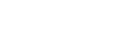# How to calculate mass, volume, center of mass of 3D solid in AutoCAD

To calculate the mass, volume, center of mass of a volumetric body in AutoCAD, there is a corresponding command button to call it is on the Details panel.Also call the command with its command-line MASS-HAR or _MASSPROP and press Enter.

After you run the command, you must select a volumetric body, then a window with the characteristics of the 3D body will appear.The first line is called Mass, but in fact it is the Volume of the selected body in mm3.

To obtain body weight, it is necessary to make some calculations, namely, multiply the value In the mass or Volume column by the density of the materials that corresponds to this solid.

To simplify and speed up this procedure, it is easier to use the appropriate application for calculating the mass of 3D body in AutoCAD.

Опубликовано Рубрики 3D modeling in AutoCADМетки calculate mass